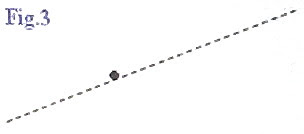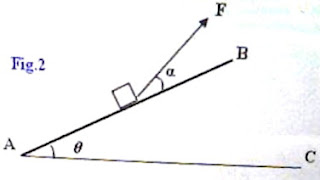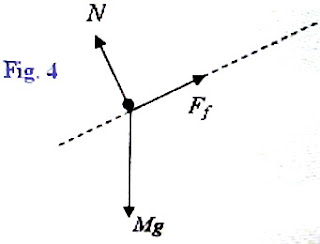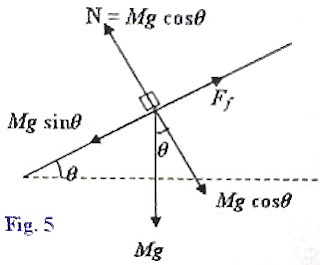## Pages

`“Life is like riding a bicycle.  To keep your balance you must keep moving.”–Albert Einstein`

## Saturday, June 27, 2009

### AP Physics B – Answer to Free Response Practice Question involving Friction

In the post dated 25th June 2009, a free-response practice question involving friction was given for the benefit of AP Physics B aspirants. As promised, I give below the answer along with the question:The figure (Fig.1) shows a block of mass M, placed on a plane surface AB inclined at an angle θ with the horizontal AC. Answer the questions relevant to the situations given below:

(a) Initially the frictional force Ff between the inclined plane and the block is sufficient to keep the block at rest. The coefficient of static friction between the incline and the block is μ. On the dot below (Fig.3) that represents the block, draw and label a free body diagram for the block in this situation.(b) Determine the magnitude of the frictional force on the block in the situation described in part (a).(c) A force is now applied on the block in a direction making an angle α (Fig.2) with the inclined plane so that the block just moves up the plane.

Determine the magnitude of the minimum force (Fmin) required for pulling the block up the plane.

(d) The inclination of the plane AB with the horizontal is made equal to 30º. When a block of mass 2 kg is placed on the incline and a gentle push is given, the block moves down the plane with constant velocity of 0.2 ms–1.

Determine the magnitude of the frictional force on the block and the coefficient of friction in this situation, assuming that g = 10 ms–2.

(a) The free body diagram is shown in Fig.4 in which Mg is the weight of the block, N is the normal force exerted on the block (by the inclined plane) and Ff is the frictional force on the block. The frictional force prevents the block from sliding down.(b) The forces acting on the block are shown in the adjoining figure (Fig.5). The component (Mgsinθ) of the weight of the body, parallel to the incline is the force trying to move the body down the plane. The normal force N is the reaction (force) opposing the component Mgcosθ of the weight of the body normal to the inclined plane. The frictional force Ff is opposite to the component Mg sinθ parallel to the plane. Since the block is at rest, the net force acting on it is zero and Ff is just sufficient to balance the component Mg sinθ (of the weight of the body).

Thus the magnitude of the frictional force on the block in this situation is Mg sinθ.

(c) When a force F is applied in the direction α as given in the question (Fig. 2), the component of this force normal to the inclined plane (Fsinα) opposes the component Mgcosθ of the weight of the body. The normal force pressing the inclined plane therefore is equal to Mgcosθ F sinα. The normal reaction (or, normal force) exerted by the inclined plane on the block is therefore given in this case by

N = Mgcosθ F sinα

The frictional force opposing the motion of the block upwards is μN = μ(Mgcosθ F sinα).

When the block just begins to move up the incline, the net force acting on it is zero. The force component trying to pull the block up the incline is Fcosα and the forces trying to oppose the motion are the weight component Mgsinθ and the frictional force = μ(Mgcosθ F sinα).

Therefore, we have Fcosα = Mgsinθ + μ(Mgcosθ F sinα)

Or, F = Mg(sinθ + μcosθ) /(cosα + μ sinα)

Since μ = tan λ where λ is the angle of friction, the above equation can be rewritten as

F = Mg [sinθ + (sinλ/cosλ) cosθ)] /[(cosα + (sinλ/cosλ) sinα)]

= Mg(sinθ cosλ + cosθ sinλ) /(cosα cosλ + sinα sinλ)

Or, F = Mg sin(θ + λ) /cos(α λ)

The pulling force will be minimum when the denominator cos(α λ) is maximum, equal to 1.

Thus the magnitude of the minimum force (Fmin) required for pulling the block up the plane is given by

Fmin = Mg sin(θ + λ)

[Note that cos(α λ) = 1 when α = λ. So the inclination of the pulling force with the inclined plane must be equal to the angle of friction].

(d) Since the block is moving down with uniform velocity, the net force acting on the block is zero. In this situation the frictional force is μMgcosθ and it acts up the plane, opposing the downward motion of the block. The component of the weight Mgsinθ of the body acts down the plane and tries to drive the body down the plane. These are thus equal in magnitude and opposite in direction.

[The velocity 0.2 ms–1 mentioned in the question should not distract you. It does not affect the answer as long as it is uniform. If its magnitude is smaller than the given value, it just means that the initial push given to the block was softer].

The magnitude of the frictional force in this situation is thus given by

Ff = Mgsinθ = 2×10× sin 30º = 10 newton.

Since μMgcosθ = Mgsinθ, the coefficient of friction is given by

μ = tanθ = tan 30º = 0.5774

You will find some useful multiple choice questions involving friction at physicsplus